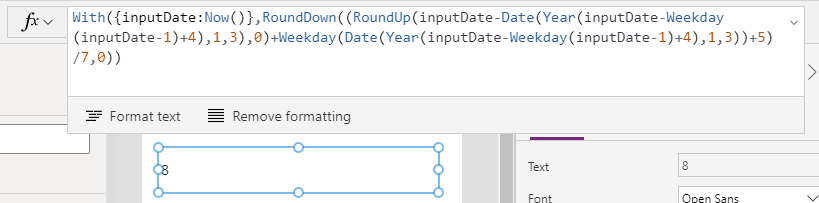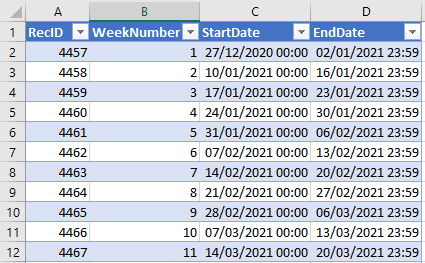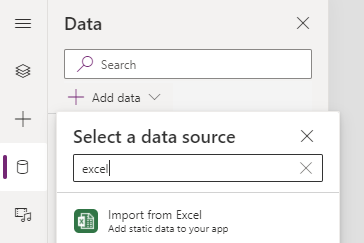# Formulas - How to hack complex date calculations

In cases where we need to carry out complex date calculations, it can be much easier to avoid the use of formula and to use static data instead. This post describes an example of how to use this technqiue to calculate week numbers.

There are often scenarios where we need to build complex formula.

This happens frequently in cases where we need to carry out date calculations. For example, there may be a requirement to display a list of all the weekends in year, or the requirement to display the first day of each quarter.

These types of calculations can be very difficult and a simple alternative not to overlook, is to build lists of static data and to import those into an app. In this post, we'll walk through an example.

## Example - How to calculate the ISO week number

Let’s suppose we want to display the week number that corresponds to a date. We can search online for formulas, and apply formulas such as the one that AUPowerapps posted here.
https://powerusers.microsoft.com/t5/Building-Power-Apps/How-to-calculate-week-number/td-p/20161

`With(    {inputDate: Now()},    RoundDown(        (RoundUp(            inputDate - Date(                Year(inputDate - Weekday(inputDate - 1) + 4),1,3            ),            0        ) + Weekday(            Date(                Year(inputDate - Weekday(inputDate - 1) + 4),1,3            )        ) + 5) / 7,        0    ))`## What are the challenges of complex formulas?

There's nothing wrong with the formula above, and it's a perfectly good technique. The main disadvantage is that it isn’t very maintainable. For apps that we develop in a team, it can be very difficult for other app builders to understand,modify and to adapt the date calculation logic.

To give an example, it's not uncommon for businesses to want to modify the date ranges. In 2021, week one starts on Janary 4th. There may be a requirement to change the start date so that week one begins on January 1st. With this change in logic, we can better label a target date of 2nd January 2021 as “Week 1 2021”, rather than “Week 53 2020”.

The problem is that it's difficult to work out the logic and to adapt the existing formula so that it takes into account the change in date range at the start and end of each year.## How to simplify this requirement by using static data

To simplify this task, a 'hack' or workaround is to build a list of static values and to import those into an app (or to make the data available through an online data connection). By using this technique, we can carry out this calculation in a more maintainable way.

The screenshot beneath shows a list of static date ranges in Excel. We can easily build this type of list by using the 'fill down' feature in Excel.We can import this spreadsheet through the data panel.Once we add the static Excel data, we can easily retrieve week number by calling the LookUp function like so.

`With({inputDate:Now()},     LookUp(WeekNumbers, inputDate >= StartDate And inputDate <=EndDate)).WeekNumber`

## Conclusion

In cases where we need to carry out complex calculations, it can be much easier and more maintainable to use static data. This post provided a demonstration by showing how to calculate week numbers.
Related posts